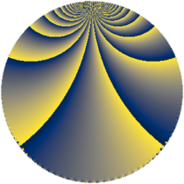# Properties

 Label 384.3.hLevel $384$ Weight $3$ Character orbit 384.h Rep. character $\chi_{384}(65,\cdot)$ Character field $\Q$ Dimension $32$ Newform subspaces $7$ Sturm bound $192$ Trace bound $5$

# Related objects

## Defining parameters

 Level: $$N$$ $$=$$ $$384 = 2^{7} \cdot 3$$ Weight: $$k$$ $$=$$ $$3$$ Character orbit: $$[\chi]$$ $$=$$ 384.h (of order $$2$$ and degree $$1$$) Character conductor: $$\operatorname{cond}(\chi)$$ $$=$$ $$24$$ Character field: $$\Q$$ Newform subspaces: $$7$$ Sturm bound: $$192$$ Trace bound: $$5$$ Distinguishing $$T_p$$: $$5$$, $$11$$

## Dimensions

The following table gives the dimensions of various subspaces of $$M_{3}(384, [\chi])$$.

Total New Old
Modular forms 144 32 112
Cusp forms 112 32 80
Eisenstein series 32 0 32

## Trace form

 $$32q + O(q^{10})$$ $$32q + 160q^{25} + 32q^{33} + 160q^{49} + 160q^{57} - 320q^{73} + 160q^{81} - 512q^{97} + O(q^{100})$$

## Decomposition of $$S_{3}^{\mathrm{new}}(384, [\chi])$$ into newform subspaces

Label Dim. $$A$$ Field CM Traces $q$-expansion
$$a_2$$ $$a_3$$ $$a_5$$ $$a_7$$
384.3.h.a $$2$$ $$10.463$$ $$\Q(\sqrt{6})$$ $$\Q(\sqrt{-6})$$ $$0$$ $$-6$$ $$0$$ $$0$$ $$q-3q^{3}+\beta q^{5}+\beta q^{7}+9q^{9}-10q^{11}+\cdots$$
384.3.h.b $$2$$ $$10.463$$ $$\Q(\sqrt{-2})$$ $$\Q(\sqrt{-2})$$ $$0$$ $$-2$$ $$0$$ $$0$$ $$q+(-1+\beta )q^{3}+(-7-2\beta )q^{9}-14q^{11}+\cdots$$
384.3.h.c $$2$$ $$10.463$$ $$\Q(\sqrt{-2})$$ $$\Q(\sqrt{-2})$$ $$0$$ $$2$$ $$0$$ $$0$$ $$q+(1+\beta )q^{3}+(-7+2\beta )q^{9}+14q^{11}+\cdots$$
384.3.h.d $$2$$ $$10.463$$ $$\Q(\sqrt{6})$$ $$\Q(\sqrt{-6})$$ $$0$$ $$6$$ $$0$$ $$0$$ $$q+3q^{3}+\beta q^{5}-\beta q^{7}+9q^{9}+10q^{11}+\cdots$$
384.3.h.e $$4$$ $$10.463$$ $$\Q(i, \sqrt{5})$$ None $$0$$ $$0$$ $$-16$$ $$0$$ $$q+\beta _{3}q^{3}-4q^{5}+(-2\beta _{1}-2\beta _{3})q^{7}+\cdots$$
384.3.h.f $$4$$ $$10.463$$ $$\Q(i, \sqrt{5})$$ None $$0$$ $$0$$ $$16$$ $$0$$ $$q-\beta _{1}q^{3}+4q^{5}+(-2\beta _{1}-2\beta _{3})q^{7}+\cdots$$
384.3.h.g $$16$$ $$10.463$$ $$\mathbb{Q}[x]/(x^{16} + \cdots)$$ None $$0$$ $$0$$ $$0$$ $$0$$ $$q+\beta _{8}q^{3}+\beta _{2}q^{5}+\beta _{1}q^{7}+(-1-\beta _{5}+\cdots)q^{9}+\cdots$$

## Decomposition of $$S_{3}^{\mathrm{old}}(384, [\chi])$$ into lower level spaces

$$S_{3}^{\mathrm{old}}(384, [\chi]) \cong$$ $$S_{3}^{\mathrm{new}}(24, [\chi])$$$$^{\oplus 5}$$$$\oplus$$$$S_{3}^{\mathrm{new}}(96, [\chi])$$$$^{\oplus 3}$$$$\oplus$$$$S_{3}^{\mathrm{new}}(192, [\chi])$$$$^{\oplus 2}$$